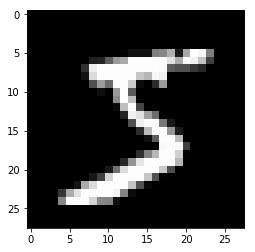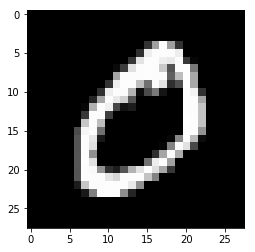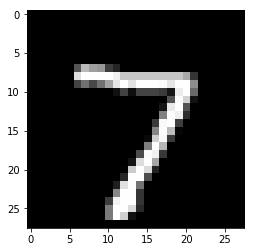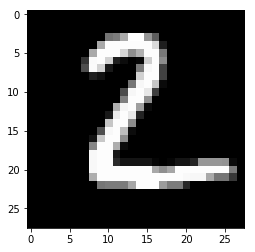## The MNIST database is a large database of handwritten digits. Each row of the dataset contains an image as a 784 element vector. The scalars represent the pixels in gray scale of a 28X28 pixel image of a digit.¶

In :
import numpy as np
import matplotlib.pyplot as plt
import csv


## Load MNIST training data file¶

In :
#Dataset has 60,000 rows and 28X28 columns
csvfile = open("mnist-train.csv")
readerobject = csv.reader(csvfile, delimiter=',')
lst = list(readerobject)
csvfile.close()


## Convert data into floating type¶

In :
y_train = np.array([x for x in lst[1:]])
x_train = np.array([x[1:] for x in lst[1:]])

y_train = y_train.astype(float)
x_train = x_train.astype(float)


## Function to plot a row of MNIST data as image¶

In :
def show_img(img):
if img.ndim == 1:
plt.figure()
img_reshaped = np.reshape(img, (28, 28))
plt.imshow(img_reshaped,cmap='gray')
plt.show()
else:
for single_img in img:
plt.figure()
single_img_reshaped = np.reshape(single_img, (28, 28))
plt.imshow(single_img_reshaped,cmap='gray')
plt.show()


## Plotting a couple of images (rows 0 and 1) using the above function¶

In :
show_img(x_train[[0,1],:])## Learn using a neural network with two hidden layers, each containing 20 neurons¶

In :
from sklearn.neural_network import MLPClassifier
from sklearn.metrics import accuracy_score

clf = MLPClassifier(hidden_layer_sizes=(20, 20))
clf.fit(X=x_train,y=y_train)

#For regression using neural network import the following
#from sklearn.neural_network import MLPRegressor

Out:
MLPClassifier(activation='relu', alpha=0.0001, batch_size='auto', beta_1=0.9,
beta_2=0.999, early_stopping=False, epsilon=1e-08,
hidden_layer_sizes=(20, 20), learning_rate='constant',
learning_rate_init=0.001, max_iter=500, momentum=0.9,
n_iter_no_change=10, nesterovs_momentum=True, power_t=0.5,
random_state=None, shuffle=True, solver='adam', tol=0.0001,
validation_fraction=0.1, verbose=False, warm_start=False)

## Checking the training accuracy¶

In :
y_true = y_train
y_pred = clf.predict(x_train)
acc = accuracy_score(y_true,y_pred)
print("Training accuracy",acc)

Training accuracy 0.9832166666666666


## Load MNIST test data file and predict a few rows¶

In :
csvfile = open("mnist-test.csv")
readerobject_test = csv.reader(csvfile, delimiter=',')
lst_test = list(readerobject_test)
csvfile.close()

y_test = np.array([x for x in lst_test[1:]])
x_test = np.array([x[1:] for x in lst_test[1:]])

y_test = y_test.astype(float)
x_test = x_test.astype(float)

indices = [0,1,2,3,4,5,6,7,8,9,10,11,12]
c_predicted = clf.predict(x_test[indices,:])
print(c_predicted)

# Use the following command to see the images
# show_img(x_test[indices,:])

[7. 2. 1. 0. 4. 1. 4. 9. 5. 9. 0. 6. 9.]


## Use the following function to compute the testing accuracy¶

In :
def testing():
csvfile = open("mnist-test.csv")
readerobject_test = csv.reader(csvfile, delimiter=',')
lst_test = list(readerobject_test)
csvfile.close()

y_test = np.array([x for x in lst_test[1:]])
x_test = np.array([x[1:] for x in lst_test[1:]])

y_test = y_test.astype(float)
x_test = x_test.astype(float)

index_to_predict = range(0,2)
c_predicted = clf.predict(x_test[index_to_predict,:])
c_predicted_probability = clf.predict_proba(x_test[index_to_predict,:])
print(c_predicted)
print(y_test[index_to_predict])
#print(c_predicted_probability)
show_img(x_test[index_to_predict,:])
print("Testing accuracy",clf.score(X=x_test,y=y_test))

In :
testing()

[7. 2.]
[7. 2.]Testing accuracy 0.9446